# 1. Introduction

Most engineers use Matlab (or its open source alternative Octave) to solve their signal processing problems. Languages such as R or Python are not immediately thought of for signal processing, although this has changed a bit in the last few years with the development of the R signal package and Python scipy.signal.

The package gsignal aims to further stimulate the use of R for signal processing tasks. It is ported from the Octave signal package, version 1.4.1 (2019-02-08). The package contains a variety of signal processing tools, such as signal generation and measurement, correlation and convolution, filtering, FIR and IIR filter design, filter analysis and conversion, power spectrum analysis, system identification, decimation and sample rate change, and windowing.

This vignette provides a brief and general overview of some of gsignal’s functions.

library(gsignal)
#>
#> Attaching package: 'gsignal'
#> The following objects are masked from 'package:stats':
#>
#>     filter, gaussian, poly

# 2. Signal generation and measurement

The function pulstran can be used to generate trains of pulses based on samples of a continuous function (which can be user-defined). The following figures show a periodic rectangular pulse, an asymmetric sawtooth pulse, a periodic Gaussian waveform, and a custom pulse train.


op <- par(mfrow = c(2, 2))

## periodic rectangular pulse
t <- seq(0, 60, 1/1e3)
d <- cbind(seq(0, 60, 2), sin(2 * pi * 0.05 * seq(0, 60, 2)))
y <- pulstran(t, d, 'rectpuls')
plot(t, y, type = "l", xlab = "", ylab = "",
main = "Periodic rectangular pulse")

## assymetric sawtooth waveform
fs <- 1e3
t <- seq(0, 1, 1/fs)
d <- seq(0, 1, 1/3)
x <- tripuls(t, 0.2, -1)
y <- pulstran(t, d, x, fs)
plot(t, y, type = "l", xlab = "", ylab = "",
main = "Asymmetric sawtooth ")

## Periodic Gaussian waveform
fs <- 1e7
tc <- 0.00025
t <- seq(-tc, tc, 1/fs)
x <- gauspuls(t, 10e3, 0.5)
ts <- seq(0, 0.025, 1/50e3)
d <- cbind(seq(0, 0.025, 1/1e3), sin(2 * pi * 0.1 * (0:25)))
y <- pulstran(ts, d, x, fs)
plot(ts, y, type = "l", xlab = "", ylab = "",
main = "Gaussian pulse")

## Custom pulse trains
fnx <- function(x, fn) sin(2 * pi * fn * x) * exp(-fn * abs(x))
ffs <- 1000
tp <- seq(0, 1, 1 / ffs)
pp <- fnx(tp, 30)
fs <- 2e3
t <- seq(0, 1.2, 1 / fs)
d <- seq(0, 1, 1/3)
dd <- cbind(d, 4^-d)
z <- pulstran(t, dd, pp, ffs)
plot(t, z, type = "l", xlab = "", ylab = "",
main = "Custom pulse")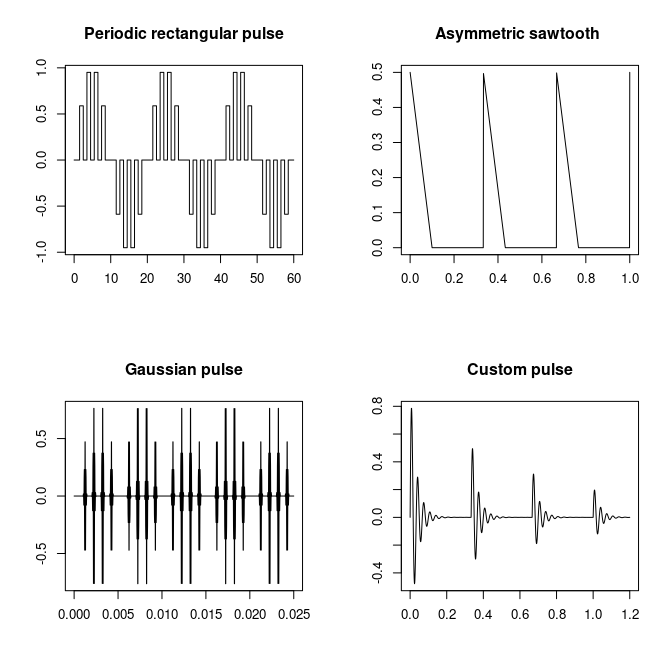par(op)

A number of waveform generating functions are available, such as chirp, cmorwavf, diric, gauspuls, gmonopuls, mexihat, meyeraux, morlet, rectpuls, sawtooth, square, and tripuls.

The function findpeaks can be used to determine (local) minima and maxima in a signal, as the following figures show.


t <- 2 * pi * seq(0, 1,length = 1024)
y <- sin(3.14 * t) + 0.5 * cos(6.09 * t) +
0.1 * sin(10.11 * t + 1 / 6) + 0.1 * sin(15.3 * t + 1 / 3)
data1 <- abs(y) # Positive values
peaks1 <- findpeaks(data1)
data2 <- y # Double-sided
peaks2 <- findpeaks(data2, DoubleSided = TRUE)
peaks3 <- findpeaks (data2, DoubleSided = TRUE, MinPeakHeight = 0.5)

op <- par(mfrow=c(1,2))
plot(t, data1, type="l", xlab="", ylab="")
points(t[peaks1$loc], peaks1$pks, col = "red", pch = 1)
plot(t, data2, type = "l", xlab = "", ylab = "")
points(t[peaks2$loc], peaks2$pks, col = "red", pch = 1)
points(t[peaks3$loc], peaks3$pks, col = "red", pch = 4)
par (op)
title("Finding the peaks of smooth data is not a big deal")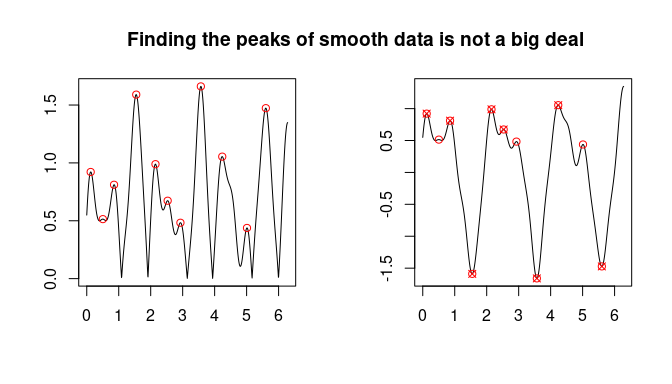t <- 2 * pi * seq(0, 1, length = 1024)
y <- sin(3.14 * t) + 0.5 * cos(6.09 * t) + 0.1 *
sin(10.11 * t + 1 / 6) + 0.1 * sin(15.3 * t + 1 / 3)
data <- abs(y + 0.1*rnorm(length(y),1))   # Positive values + noise
peaks1 <- findpeaks(data, MinPeakHeight=1)
dt <- t-t
peaks2 <- findpeaks(data, MinPeakHeight=1, MinPeakDistance=round(0.5/dt))
op <- par(mfrow=c(1,2))
plot(t, data, type="l", xlab="", ylab="")
points (t[peaks1$loc],peaks1$pks,col="red", pch=1)
plot(t, data, type="l", xlab="", ylab="")
points (t[peaks2$loc],peaks2$pks,col="red", pch=1)
par (op)
title(paste("Noisy data may need tuning of the parameters.\n",
"In the 2nd example, MinPeakDistance is used\n",
"as a smoother of the peaks"))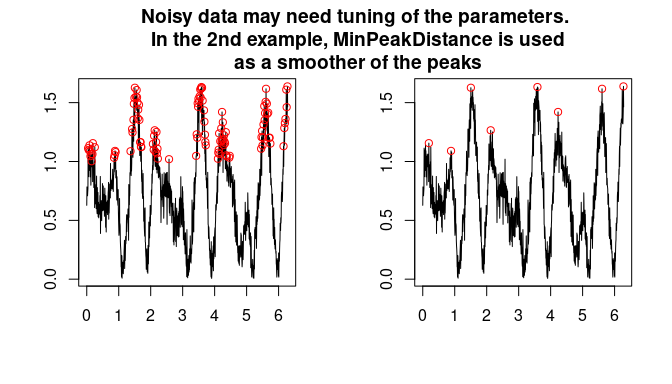# 3. Filter Design

The gsignal package contains functions for designing lowpass, highpass, bandpass, and bandstop filters. Both Finite Impulse Response (FIR) and Infinite Impulse Response (IIR) filters can be designed. The freqz function displays the frequency response’s magnitude and phase of the filter.

## FIR filters

A FIR filter is a filter whose impulse response settles to zero in finite time. This is in contrast to IIR filters, which have internal feedback causing them to have an infinitely long impulse response (although usually decaying).

For causal discrete-time FIR filters the output is a weighted sum of the most recent input values. Compared to IIR filters, advantages of FIR filters are they are inherently stable (because there is no feedback that propagates indefinitely), and that they have linear phase (constant across frequencies). The main disadvantage is that they require more computation time to obtain sharp transition bands.

The package gsignal contains various methods to design digital FIR filters. The functions fir1, fir2, and kaiserord use the windowing method, in which a window is applied to the truncated inverse Fourier transform of the filter’s frequency response. The function firls is an extension of the fir1 and fir2 functions that uses a least-squares approach to minimize errors between the specified and the actual frequency response over sub-bands of the frequency range. The Parks-McClellan method using the Remez exchange algorithm for finding an optimal equiripple set of filter coefficients is used by the remez function. The cl2bp function allows designing FIR filters without explicitly defining the transition bands for the magnitude response.

Below are some examples of FIR filter design. The magnitude and the phase of the filter’s frequency response are plotted by the function freqz.


## FIR filter design by windowing

# low-pass filter 10 Hz
fs = 256
h <- fir1(40, 10/ (fs / 2), "low")
freqz(h, fs = fs)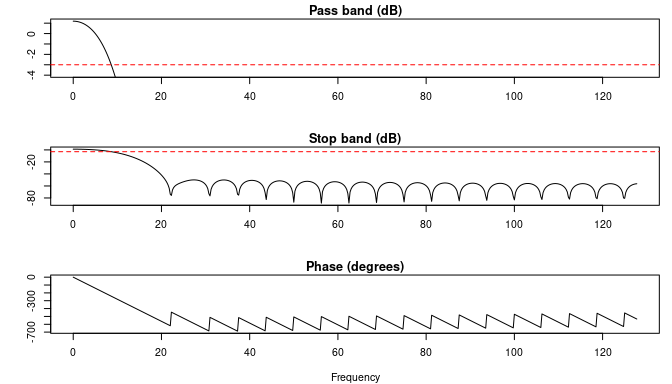# observe the effect of filter length
h <- fir1(80, 10/ (fs / 2), "low")
freqz(h, fs = fs)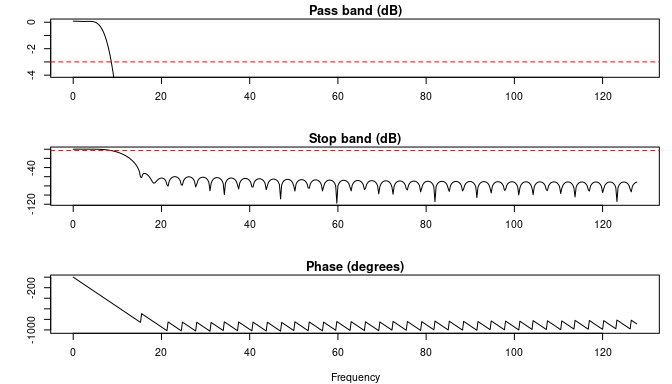# fir2 allows specifying arbitrary frequency responses
f <- c(0, 0.3, 0.3, 0.6, 0.6, 1)
m <- c(0, 0, 1, 1/2, 0, 0)
fh <- freqz(fir2(100, f, m))
op <- par(mfrow = c(1, 2))
plot(f, m, type = "b", ylab = "magnitude", xlab = "Frequency")
lines(fh$w / pi, abs(fh$h), col = "blue")
legend("topright", legend = c("specified", "actual"), lty = 1,
pch = c(1, NA), col = c("black", "blue"))
# plot in dB:
plot(f, 20*log10(m+1e-5), type = "b", ylab = "dB", xlab = "Frequency")
lines(fh$w / pi, 20*log10(abs(fh$h)), col = "blue")
par(op)
title("specify arbitrary frequency responses with fir2")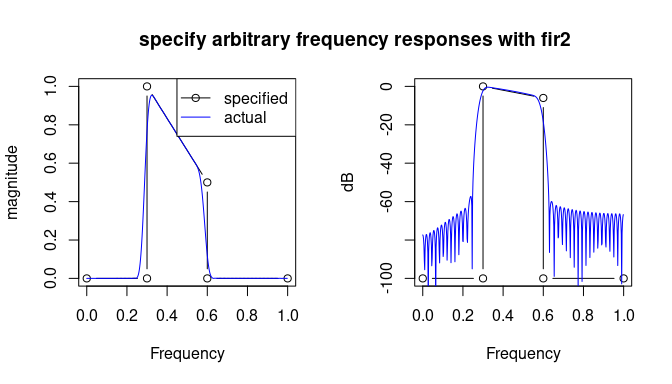## 50 Hz notch filter with remez
fs <- 200
nyquist <- fs / 2
f <- c(0, 48.5 / nyquist, 49.5 / nyquist, 50.5 / nyquist, 51.5 / nyquist, 1)
a <- c(1, 1, 0, 0, 1, 1)
h <- remez(200, f, a)
freqz(h, fs = fs)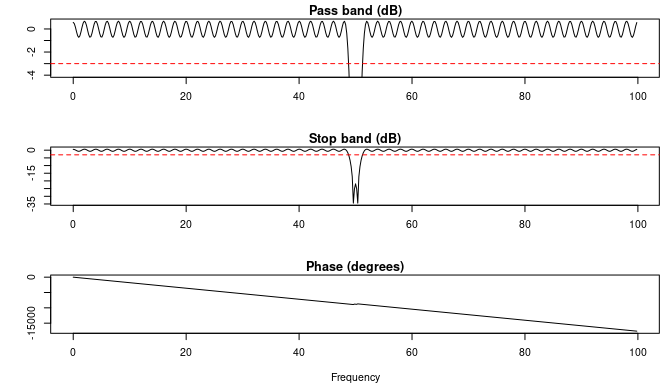### Compensating for filter delay in FIR filters

Filtering causes a delay because weighted samples in the past are used. FIR filters have a linear phase, so in the time domain this delay is constant, namely $$N / 2$$, where $$N$$ is the filter length (or ‘number of taps’). The function grpdelay can be used to calculate the group delay; see Figure (a) below. Because phase is linear, it is easy to to compensate for the filter delay as shown in Figure (b) below.

op <- par(mfrow = c(2, 1))
# design the filter
fs = 256
h <- fir1(40, 30/ (fs / 2), "low")

# group delay is constant at N/2
gd <- grpdelay(h)
plot(gd, ylim = c(0, 40),
main = paste("(a) Group delay for FIR filters is constant\n",
"(here 40 / 2 = 20)"))

# filter electrocardiogram data with added noise
data(signals)
npts <- nrow(signals)
ecg <- signals$ecg + 1000 * runif(npts) time <- seq(0, 10, length.out = npts) plot(time, ecg, type = "l", main = "(b) Example ECG signal", xlab = "Time", ylab = "", xlim = c(0,2)) title(ylab = expression(paste("Amplitude (", mu, "V)")), line = 2) f1 <- filter(h, ecg) lines(time, f1, col = "red", lwd = 2) delay <- mean(gd$gd)
f2 <- c(f1[(delay + 1):npts], rep(NA, delay + 1))
lines(time, f2, col = "blue", lwd = 2)
legend("topright", legend = c("Original", "Filtered", "Corrected"),
lty = 1, lwd = c(1, 2, 2), col = c("black", "red", "blue"))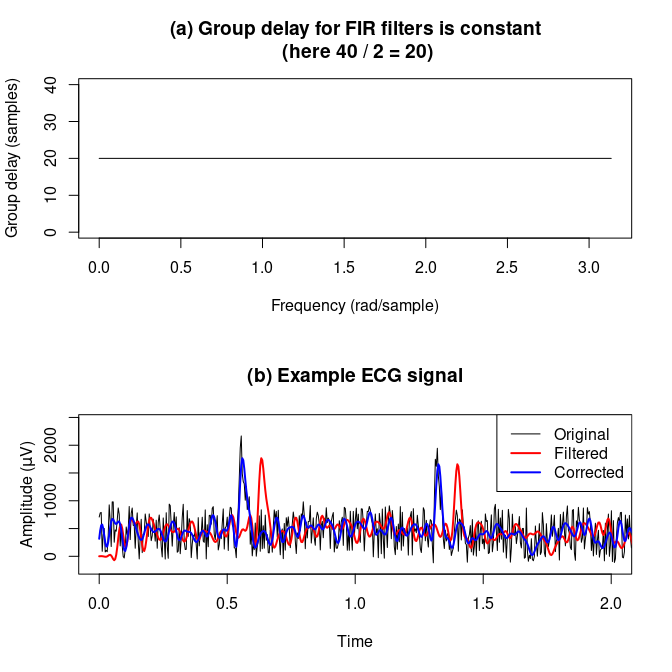par(op)

## IIR filters

Infinite Impulse Response, or recursive, filters are an efficient way of achieving a long impulse response by not only using past input samples, but also past output samples. Hence, an element of feedback (recursion) is used. IIR filters are specified by a set of feedback coefficients (usually termed $$a$$), in addition to feedforward coefficients ($$b$$) as used in FIR filters.

Advantages of IIR filters compared to FIR filters are related to their efficiency in implementation. IIR filters usually require (much) fewer filter coefficients, implying a correspondingly fewer number of calculations. On the other hand, the impulse response of IIR filters does not always decay to zero, which may result in filter instability (see the example below). In addition, the phase of IIR filters is not linear but frequency dependent. Forward and reverse filtering (filtfilt) results in zero phase at the expense of additional computing time (there is no free lunch).

Some important types of IIR filters are:

1. Butterworth filters have frequency response that is as flat as possible in the passband (function butter);
2. Chebyshev filters are IIR filters having a steeper roll-off than Butterworth filters, and either have a passband ripple (Type I - function cheby1), or a stopband ripple (Type II - function cheby2);
3. Elliptic filters with equalized ripple (equiripple) behavior in both the passband and the stopband (function ellip);
4. (Analog) Bessel filters with a maximally linear phase response.

The following figure compare the frequency responses of (a) 5th order Butterworth and Chebyshev filters, (b) 5th order Butterworth and elliptic filters, and (c) type I and type II Chebyshev filters.


op <- par(mfrow = c(3,1))

# compare Butterworth and Chebyshev filters.
bfr <- freqz(butter(5, 0.1))
cfr <- freqz(cheby1(5, .5, 0.1))
plot(bfr$w, 20 * log10(abs(bfr$h)), type = "l", ylim = c(-80, 0),
xlab = "Frequency (rad)", ylab = c("dB"),
main = "(a) Butterworth and Chebyshev")
lines(cfr$w, 20 * log10(abs(cfr$h)), col = "red")
legend("topright", legend = c("5th order Butterworth", "5th order Chebyshev"),
lty = 1, col = c("black", "red"))

# compare Butterworth and elliptic filters.
efr <- freqz(ellip(5, 3, 40, 0.1))
plot(bfr$w, 20 * log10(abs(bfr$h)), type = "l", ylim = c(-80, 0),
xlab = "Frequency (rad)", ylab = c("dB"),
main = "(b) Butterworth and Elliptic")
lines(efr$w, 20 * log10(abs(efr$h)), col = "red")
legend ("topright", legend = c("5th order Butterworh", "5th order Elliptic"),
lty = 1, col = c("black", "red"))

# compare type I and type II Chebyshev filters.
c1fr <- freqz(cheby1(5, .5, 0.1))
c2fr <- freqz(cheby2(5, 20, 0.1))
plot(c1fr$w, 20 * log10(abs(c1fr$h)), type = "l", ylim = c(-80, 0),
xlab = "Frequency (rad)", ylab = c("dB"),
main = "(c) Type I and II Chebyshev")
lines(c2fr$w, 20 * log10(abs(c2fr$h)), col = "red")
legend ("topright", legend = c("5th order Type I", "5th order Type II"),
lty = 1, col = c("black", "red"))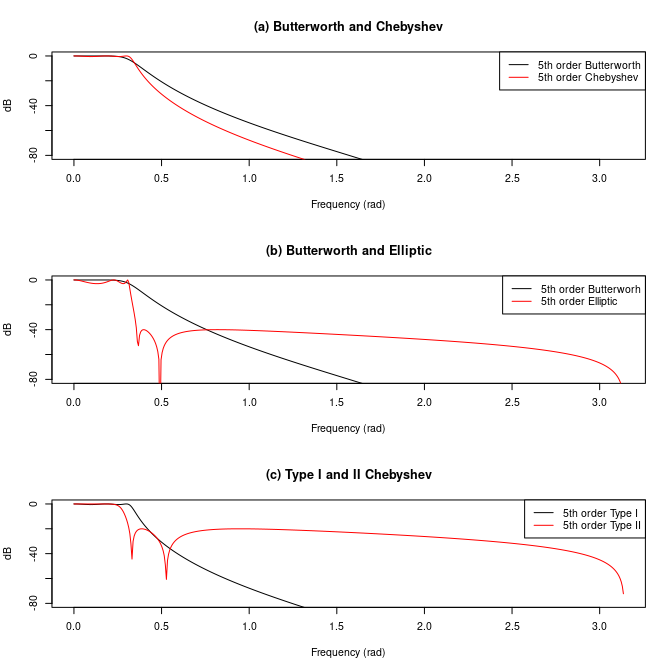par(op)

### Numerical precision and stability

Using IIR filter coefficients $$b, a$$ can cause numerical problems. Therefore, IIR filter design functions in gsignal have an output parameter, allowing the filter coefficients to be returned in one of three forms:

• Arma, a list containing the moving average polynomial (feedforward) coefficients $$b$$, and the autoregressive (recursive, feedback) coefficients $$a$$;
• Zpg, a list containing the coefficients in zero-pole-gain form
• Sos, a list of series second order sections (biquads)

Although the Arma form is default for compatibility reasons, the use of Sos and the accompanying filtering function sosfilt is generally preferred.

A second issue that may occur when using IIR filters, is instability. This may be the result of numerical rounding errors or because too many filter coefficients were used. Pole-Zero Analysis can be useful here. A filter is stable if its impulse response $$h(n)$$ decays to 0 when $$n$$ increases. In terms of poles and zeros, this is true if all of the filter’s poles are inside the unit circle in the $$z$$-plane (Smith, J.O. (2012)). The package gsignal offers the function zplane that displays a filter’s poles and zeros in the complex $$z$$-plane, as the following figure illustrates. In the figure the ’0’s represent the zeros, and the ’X’s the poles.


n <- layout(matrix(c(1, 2, 3, 3), nrow = 2, byrow = TRUE))
stable <- butter(3, 0.2, "low", output = "Zpg")

instable <- stable
instable$p <- instable$p - 2

zplane(stable, main = "Stable")
zplane(instable, main = "Instable")

t <- seq(0, 1, len = 100)
x <- sin(2* pi * t * 2.3) + 0.5 * rnorm(length(t))
z1 <- filter(stable, x)
z2 <- filter(instable, x)
plot(t, x, type = "l", xlab = "", ylab = "")
lines(t, z1, col = "green", lwd = 2)
lines(t, z2, col = "red")
legend("bottomleft", legend = c("Original", "Stable", "Instable"),
lty = 1, col = c("black", "green", "red"), ncol = 3)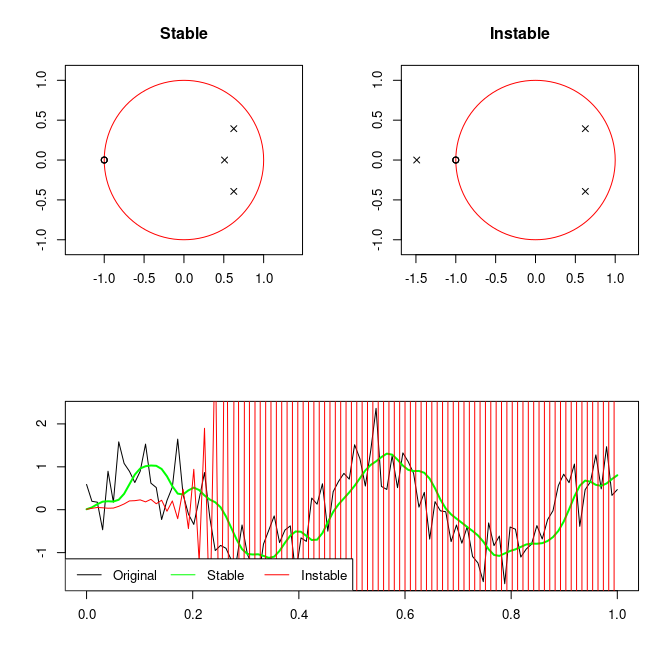par(op)

### Compensating for filter delay in IIR filters

Because the phase of the frequency response of IIR filters is not linear, the filter delay cannot be easily compensated for as in the FIR case. Recall that the 40-tap 30 Hz low-pass FIR filter used above for filtering the ECG signal had a linear phase and a constant delay of 20 samples. If a 5th order elliptic low-pass filter at 30 Hz is used, it can easily be seen that its phase is not linear (Figure (a) below), and hence the filter delay is dependent of frequency (Figure (b) below). Note that the group delay is defined to be the negative first derivative of the filter’s phase response.

op <- par(mfrow = c(2, 1))
ell <- ellip(5, 0.1, 60, 30/(fs/2), "low")
ellf <- freqz(ell, fs = fs)
argh <- Arg(ellf$h) argh[which(is.na(argh))] <- 0 phase <- unwrap(argh) plot(ellf$w, phase, type = "l", xlab = "Frequency (Hz)", ylab = "Phase",
main = paste("30 Hz 5th order elliptical low-pass IIR filter\n",
"phase response is not linear"))
gd <- grpdelay(ell, fs = fs)
#> Warning in grpdelay.default(filt$b, filt$a, ...): setting group delay to 0 at
#> singularity
plot(gd, main = paste("group delay depends on frequency\n",
"mean:", round(mean(gd$gd), 1), "samples"))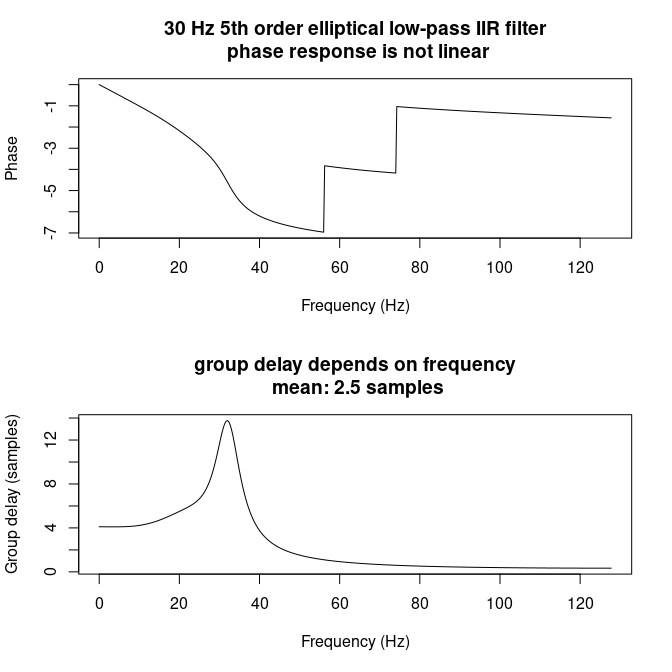par(op) This means that the filter delay cannot be compensated for in the same way as for the FIR filter. An alternative is to use the function filtfilt, which applies forward and backward filtering and thus compensates for the delay, as shown in the figure below.  f <- filter(ell, ecg) ff <- filtfilt(ell, ecg) plot(time, ecg, type = "l", xlab = "Time", ylab = "", xlim = c(0,2)) title(ylab = expression(paste("Amplitude (", mu, "V)")), line = 2) lines(time, f, col = "red", lwd = 2) lines(time, ff, col = "blue", lwd = 2) legend("topright", legend = c("Original", "filter()", "filtfilt()"), lty = 1, lwd = c(1, 2, 2), col = c("black", "red", "blue"))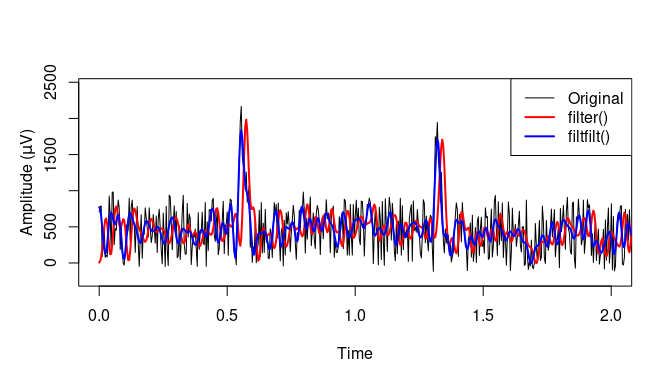# 4. Filtering and Convolution The most straightforward way to implement a digital filter is by convolving the input signal with the filter’s impulse response. The package gsignal contains several functions for convolution. The function conv returns the 1-D convolution of two vectors a and b in 3 ‘shapes’; “full”, for which the output vector has a length equal to length(a) + length(b) - 1; “valid”, which only returns the central part of the convolution with an output length of length(a); or “valid”, which returns only those parts of the convolution that are computed without the zero-padded edges (output length max(length(a) - length(b) + 1, 0)). For example:  u <- rep(1, 3) v <- c(1, 1, 0, 0, 0, 1, 1) conv(u, v, "full") #>  1 2 2 1 0 1 2 2 1 conv(u, v, "same") #>  1 0 1 conv(u, v, "valid") #> NULL conv(v, u, "valid") #>  2 1 0 1 2 Two-dimensional convolution of two matrices can be computed by the conv2 function. In this case, the size of the output matrix is nrow(A) + nrow(B) - 1 by ncol(A) + ncol(B) - 1 for “full” convolution, nrow(A) by ncol(A) for “same”, and max(nrow(A) - nrow(B) + 1, 0) by max(ncol(A) - ncol(B) + 1, 0) for “valid”. The function conv2 is implemented in C++ for speed. For long series convolution may be sped up by making use of the fact that convolution in the time domain is equivalent to multiplication in the frequency domain. Thus, the two series may be padded to the same length, converted to the frequency domain by FFT, multiplied point-wise, and transformed back to the time domain. The function cconv uses this approach. However, if one series is much longer than the other (as in typical filtering operations), zero-padding the shorter series to the length of the longer series may not be the most efficient method. In such cases, even faster methods like the overlap-add method used by the function fftconv may be useful. That’s the theory at least…  short <- runif(20L) long <- runif(1000L) # convolve two long series ll <- microbenchmark::microbenchmark(conv(long, long), cconv(long, long), fftconv(long, long)) plot1 <- ggplot2::autoplot(ll) #> Coordinate system already present. Adding new coordinate system, which will replace the existing one. # convolve a short and a long series sl <- microbenchmark::microbenchmark(conv(short, long), cconv(short, long), fftconv(short, long)) plot2 <- ggplot2::autoplot(sl) #> Coordinate system already present. Adding new coordinate system, which will replace the existing one. gridExtra::grid.arrange(plot1, plot2, nrow = 2, ncol = 1)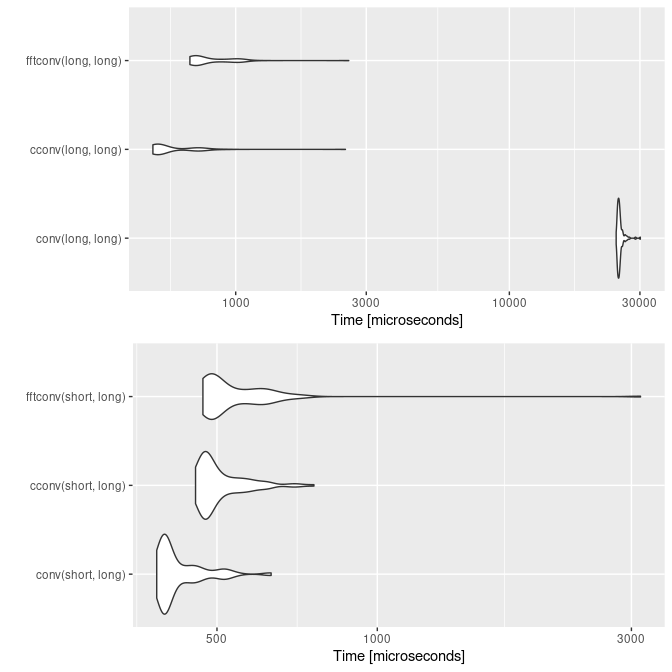One-dimensional Filtering in gsignal is performed by the function filter. It is a direct form II transposed implementation in C++ of the standard linear time-invariant difference equation $\sum_{k=0}^{N} a(k+1) y(n-k) + \sum_{k=0}^{M} b(k+1) x(n-k) = 0; 1 \le n \le length(x)$ If a matrix is passed to filter, its columns are filtered. Two-dimensional filtering can be done using filter2. THe function fftfilt uses the overlapp-add method and FFT convolution for speed (but your mileage may vary), and filtfilt uses forward and backward filtering to avoid filter delay, as used above. If numerical stability of filter coefficients is an issue, filter design using series second order sections and filtering with the function sosfilt may be used. ## Filtering long series in chunks The functions filter and sosfilt can retain the filter state in order to process long data series in chunks. The following piece of code shows how this is done. A long series is split into two parts, which are processed sequentially. In the first call to filter, the final conditions zf are asked to be returned after filtering the first part, which are then passed to the second call to filter as the initial conditions zi for the second part of the series. The two filtered parts can then be concatenated for form the entire filtered series without discontinuities.  N <- 10000L long <- runif(N) part1 <- long[1:(N / 2)] part2 <- long[((N / 2) + 1):N] b <- c(2, 3) a <- c(1, 0.2) y <- filter(b, a, long) y1 <- filter(b, a, part1, 'zf') y2 <- filter(b, a, part2, y1$zf)
yy <- c(y1$y, y2$y)
all.equal(y, yy)
#>  TRUE

## Using initial conditions to avoid filter startup effects

Initial conditions can also be used to set the initial state of the filter so that the output starts at the same value as the first element of the signal to be filtered. The initial conditions for the filter can be computed using the functions filter_zi, or filtic, as shown in the following example.


t <- seq(-1, 1, length.out =  201)
x <- (sin(2 * pi * 0.75 * t * (1 - t) + 2.1)
+ 0.1 * sin(2 * pi * 1.25 * t + 1)
+ 0.18 * cos(2 * pi * 3.85 * t))
h <- butter(3, 0.05)
zi <- filter_zi(h)
## alternatively, use:
## lab <- max(length(h$b), length(h$a)) - 1
## zi <- filtic(h, rep(1, lab), rep(1, lab))
z1 <- filter(h, x)
z2 <- filter(h, x, zi * x)
plot(t, x, type = "l", xlab ="", ylab = "")
lines(t, z1, col = "red")
lines(t, z2\$y, col = "green")
legend("bottomright", legend = c("Original signal",
"Filtered without initial conditions",
"Filtered with initial conditions"),
lty = 1, col = c("black", "red", "green"))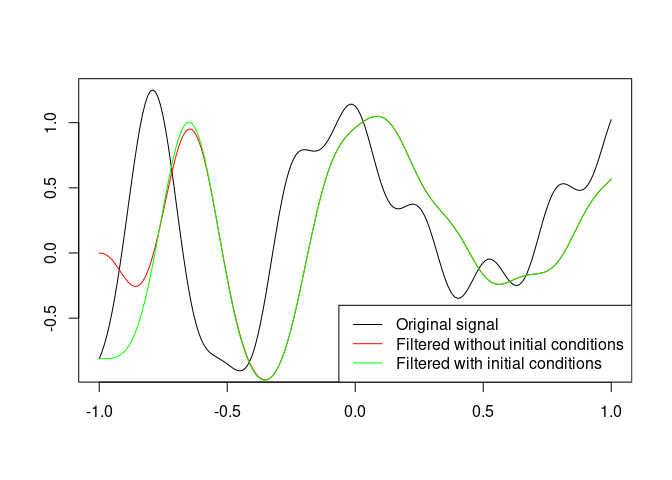# 5. Power spectrum analysis

The power spectral density (PSD) of a time series describes the distribution of power (variance) into frequency components composing that signal. The Fourier Transform is a nonparametric method of decomposing a signal into its frequency spectrum. The functions fft and ifft compute the Discrete Fourier Transform with a fast algorithm, the FFT. A parametric alternative for autoregressive (AR) models is available through the functions ar_psd, pburg, or pyulear. The following figure shows how both FFT- and AR-based methods can discover the periodicities of 5 and 12 Hz in a noisy signal.


op <- par(mfrow = c(3, 1))
fs <- 200
nsecs <- 10
lx <- fs * nsecs
t <- seq(0, nsecs, length.out =  lx)
# signal of 5 Hz + 12 Hz + noise
x <- (sin(2 * pi * 5 * t)
+ sin(2 * pi * 12 * t)
+ runif(lx))
plot(t, x, type = "l", xlab = "Time (s)", ylab = "", main = "Original signal")
pw <- pwelch(x, window = lx, fs = fs, detrend = "none")
plot(pw, xlim = c(0, 20), main = "PSD estimate using FFT")

py <- pyulear(x, 30, fs = fs)
plot(py, xlim = c(0, 20), main = "PSD estimate using Yule-Walker")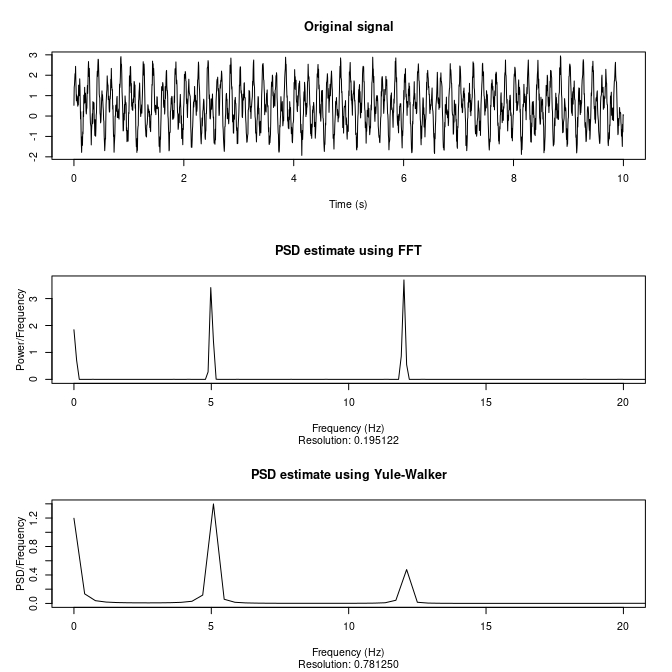par(op)

## Welch’s method

Welch (1967) proposed a method to estimate the power spectrum that reduces the variance of the spectrum (ath the expense of decreasing frequency resolution - remember, there is no free lunch) by splitting the signal into (usually) overlapping segments and windowing each segment, for instance by a Hamming window. The periodogram is then computed for each segment, and the squared magnitude is computed, which is then averaged for all segments. The spectral density is the mean of the modified periodograms, scaled so that area under the spectrum is the same as the mean square of the data. In case of multivariate signals, cross-spectral density, phase, and coherence are also returned. The input data can be demeaned or detrended, overall or for each segment separately.

The following figure shows two signals, a sine and a cosine of 5 Hz with noise added. Sines and cosines are the same waveforms, the only difference being that a cosine leads the sine wave by an amount of 90$$^{\circ}$$ ($$\pi / 2$$ radians). Hence, a plot of the PSD shoulld show identical shapes for both signals, but a phase difference at $$\pi / 2$$ radians should be visible at 5 Hz, which should also produce a high magnitude squared coherence (near 1) at that frequency (coherence reflects constant phase differences).


op <- par(mfrow = c(3, 1))
fs <- 200
nsecs <- 100
lx <- fs * nsecs
t <- seq(0, nsecs, length.out =  lx)
# sine and cosine of signal of 5 Hz noise
x1 <- cos(2 * pi * 5 * t) + runif(lx)
x2 <- sin(2 * pi * 5 * t) + runif(lx)
x <- cbind(x1, x2)
pw <- pwelch(x, fs = fs)
plot(pw, plot.type = "spectrum", yscale = "dB", xlim = c(0, 50),
main = "A sine and a cosine of 5 Hz have the same PSD")
legend("topright", legend = c("Cosine", "Sine"), lty = 1:2, col = 1:2)
rect(3, -35, 7, -4, border = "red", lwd = 3)
plot(pw, plot.type = "phase", xlim = c(0, 50),
main = expression(bold(paste("but differ ", pi/2, " radians in phase at 5 Hz"))))
rect(3, -pi, 7, pi, border = "red", lwd = 3)
plot(pw, plot.type = "coherence", xlim = c(0, 50),
main = "leading to coherence ~ 1 at 5 Hz")
rect(3, 0, 7, 1, border = "red", lwd = 3)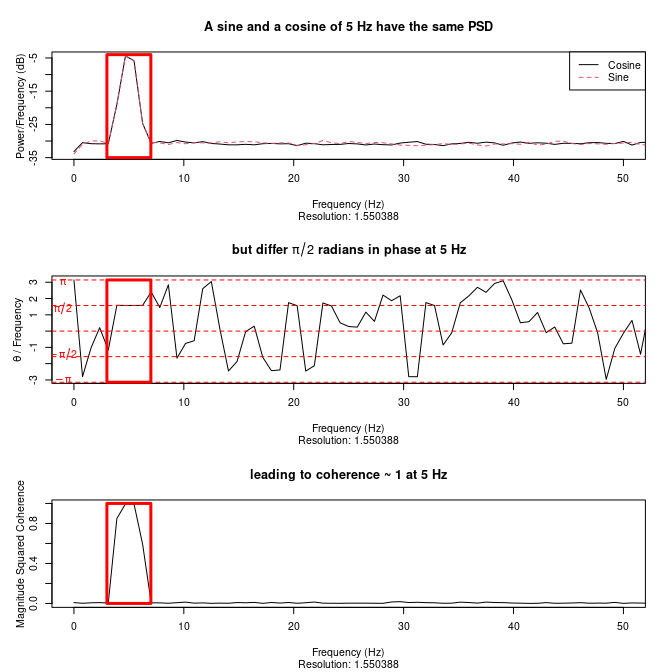par(op)

## Time-frequency analysis

If your signal’s frequency content changes over time, the power spectrum is of limited use. You might then want to use some form of time-frequency analysis. Specialized R packages exist for wavelet analysis capable of doing time-frequency analysis, such as wavelets, Rwave, and waveslim. gsignal does contain a basic function for computing the discrete wavelet transform (dwt), but is not otherwise specialized for wavelet analysis.

Another way of decomposing a signal both in time and frequency is the Short-Term Fourier Transform, which can be calculated by the function stft. Here, the data to be transformed is broken up into (overlapping) chunks. Each chunk is then Fourier transformed, and added to a record of magnitude and phase for each point in time and frequency. Alternatively, the spectrogram (specgram) in essence does the same - the spectogram is the squared magnitude of the STFT of the signal.

The following figure represents the spectrogram of a chirp signal, which is a signal in which the frequency changes with time. By default, the specgram and stft function produce grayscale plots, but here it is shown how other color palettes can be used.


op <- par(mfrow = c(2, 1))

jet <- grDevices::colorRampPalette(c("#00007F", "blue", "#007FFF", "cyan", "#7FFF7F",
"yellow", "#FF7F00", "red", "#7F0000"))
sp <- specgram(chirp(seq(0, 5, by = 1/8000), 200, 2, 500, "logarithmic"), fs = 8000)
plot(sp, col = jet(20))

c2w <- grDevices::colorRampPalette(colors = c("red", "white", "blue"))
plot(sp, col = c2w(50))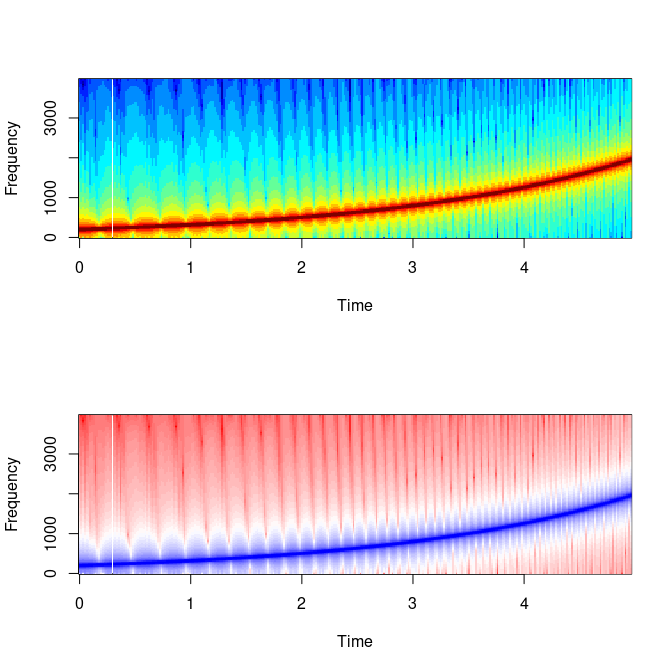par(op)

# 6. Miscellaneous functions

The package also contains many other functions that may be useful in various signal processing applications.

• Windowing functions. Window functions are usually bell-shaped functions (although rectangular and triangular shapes are also used), which are multiplied by the signal in order to taper the ends of the signal to zero to reduce spectral leakage. For instance, the pwelch function described above uses a window function (default Hamming) before FFT-ing the segments. The window functions available in gsignal are:

• Modified Bartlett-Hann (barthannwin)
• Bartlett (triangular - bartlett)
• Blackman window (blackman)
• Blackman-Harris (blackmanharris)
• Blackman-Nuttall (blackmannuttall)
• Bohman (bohmanwin)
• Boxcar (rectangular - boxcar)
• Chebyshev (chebwin)
• Flat top (flattopwin)
• Gaussian (gausswin)
• Hamming (hamming),
• Hanning (hanning - alias hann)
• Kaiser (kaiser)
• Nuttall (nuttallwin)
• Parzen (parzenwin)
• Rectangular (rectwin)
• Triangular (triang)
• Tukey (tukeywin)
• Ultraspherical (ultrwin)
• Welch (welchwin)
• Resampling functions. Up- or downsampling signals by an integer factor can be done with upsample and downsample. The function decimate and interp also down- or upsample by an integer factor, but allows specifying an IIR or FIR filter to be applied before resampling to avoid aliasing. The more general function resample allows changing the sampling rate by arbitrary factors, and also takes an impulse response of a FIR filter as an optional argument (if not specified resample will design an optimal filter for you). The function upfirdn performs three operations; it first upsamples the input signal by inserting zeros, then applies a FIR filter, and finally downsamples the signal by throwing away samples. Because this function uses a polyphase implementation for filtering, it is often faster than when filter is used (the resample function uses upfirdn for exactly that reason).

• Other functions. Various other functions not specifically addressed in this vignette are also included, which can be used for a variety of signal processing operations, such as padding data (pad, prepad, postpad), detrending data (detrend), unwrapping frequency response phase (unwrap), data transformations (cceps, czt dct, dct2, dctmtx, dftmtx, dst, dwt, fftshift, fht, fwht, hilbert, idct, idct2, idst, ifht, ifwht, rceps), polynomial analysis (poly, polystab, residue, residued, residuez, polyreduce, mpoles). Some helper functions (cplxpair, cplxreal, db2pow, pow2db, fftshift, ifftshift, digitrevorder), and other utilities are also included (e.g., buffer, shiftdata, unshiftdata, peak2peak, peak2rms, rms, rssq, clustersegment, fracshift, marcumq, primitive, sampled2continuous, schtrig, upsamplefill, wkeep, zerocrossing)